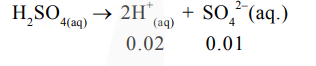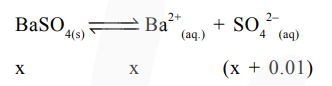# The solution of CdSO4, in water is

Question:

The solubility of $\mathrm{CdSO}_{4}$ in water is $8.0 \times 10^{-4} \mathrm{~mol} \mathrm{~L}^{-1}$. Its solubility in $0.01 \mathrm{M}$ $\mathrm{H}_{2} \mathrm{SO}_{4}$ solution is_________ $\times 10^{-6} \mathrm{~mol} \mathrm{~L}^{-1}$.

(Round off to the Nearest integer) (Assume that solubility is much less than $0.01 \mathrm{M}$ )

Solution:

In pure water,

$\mathrm{K}_{\mathrm{sp}}=\mathrm{S}^{2}=\left(8 \times 10^{-4}\right)^{2}$

$=64 \times 10^{-8}$

In $0.01 \mathrm{M} \mathrm{H}_{2} \mathrm{SO}_{4}$$\mathrm{K}_{\mathrm{sp}}=\mathrm{x}(\mathrm{x}+0.01)$

$=64 \times 10^{-8}$

$\mathrm{x}+0.01 \cong 0.01 \mathrm{M}$

So, $x(0.01)=64 \times 10^{-8}$

$x=64 \times 10^{-6} \mathrm{M}$